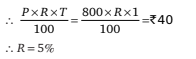# Mathematical Aptitude - Interest

>>>>>>>>Interest

• A

Rs 705• B

Rs 615• C

Rs 676• D

Rs 625• Option : D
• Explanation :• A

13.5 years• B

27 years• C

9 years• D

18 years• Option : D
• Explanation : Let amount be Rs ‘A’
It becomes double = 2A in 9 years.
In next 9 years it again double i.e., becomes 2 × 2A = 4A
So in 9 + 9 = 18 years the amount becomes four times itself.

• A

4%• B

5%• C

8%• D

None of the above• Option : B
• Explanation : Simple interest for each year = 40/2 = Rs 20
Compound Interest for first year is Rs 20 and for second year = 41 – 20 = 21
Thus Rs 20 becomes Rs 21, thus rate of interest can be calculated
P = Rs 20, SI = Rs 1, T = 1 year
So R = SI × 100/P × T = 1 × 100/20 × 1 = 5%

• A

5%• B

6%• C

7%• D

None of the above• Option : A
• Explanation : 4 years – 3 years = 1 year
Thus, we can say that Rs 800 becomes Rs 840 in 1 year.
∴ Interest = Rs 840 – Rs 800 = Rs 40
For 1 year time period, Simple Intesest = Compound Interest• A

8 years• B

16 years• C

18 years• D

24 years• Option : C
• Explanation : 4 times in 6 years
So 16 times in 12 years
And 64 times in 18 years.
Related Quiz.
Interest